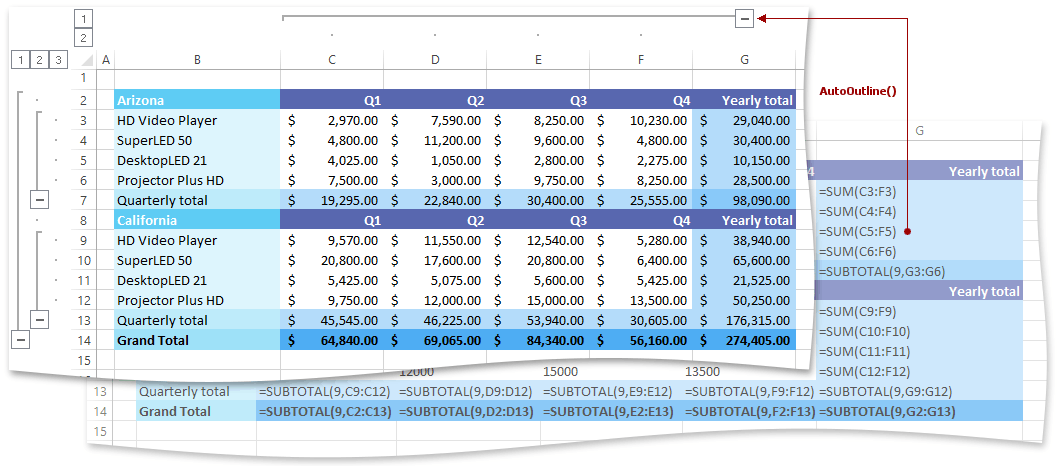.NET Framework 4.5.2+

# How to: Outline Data Automatically

The example below demonstrates how to outline data automatically using the Worksheet.AutoOutline method.

The Worksheet.AutoOutline method uses worksheet formulas to determine how to group data. Thus, make sure that the worksheet you wish to outline contains summary rows or columns with formulas based on which the outline will be created. If you did not specify any summary formulas, the data will not be grouped.

To remove an outline for the specified worksheet, use the Worksheet.ClearOutline method.

``````// Outline the data automatically based on the summary formulas.
worksheet.AutoOutline();
``````

The image below shows the source worksheet to which the Worksheet.AutoOutline method is applied, and the resulting document with the corresponding outlines of rows and columns (the workbook is opened in Microsoft® Excel®).